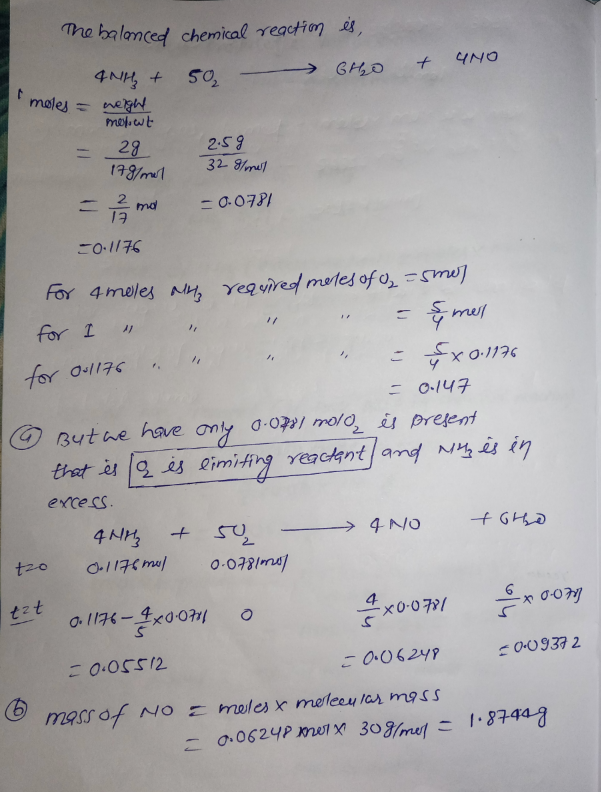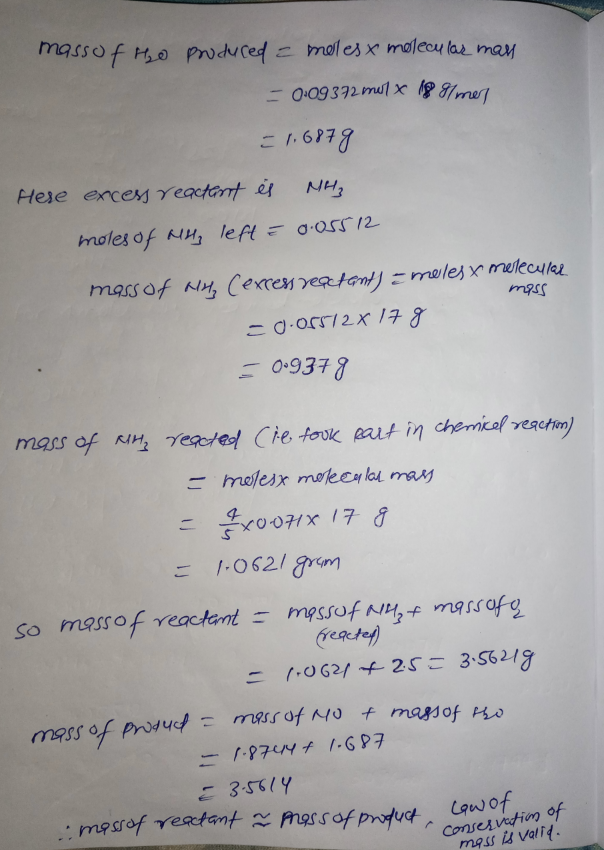In: Chemistry

# One of the steps in the commercial process for converting ammonia to nitric acid is the...

One of the steps in the commercial process for converting ammonia to nitric acid is the conversion of NH3 to NO:
NH.3 (g) + (g) NO (g) H20(g)
In a certain experiment, 2.00 grams of ammonia reacts with 2.50 grams of o2. What is the limiting reagent? How many grams of NO and H2O form? How many grams of the excess reactant remain after the limiting reactant is completely consumed? Show by your calculations that the Law of Conservation by mass is not violated.

## Solutions

##### Expert Solution## Related Solutions

##### One of the steps in the commercial process for converting ammonia to nitric acid is the...
One of the steps in the commercial process for converting ammonia to nitric acid is the conversion of NH3 to NO: 4NH3(g)+5O2(g)→4NO(g)+6H2O(g) In a certain experiment, 1.20 g of NH3 reacts with 2.20 g of O2. a) How many grams of NO and of H2O form? Enter your answers numerically separated by a comma. b) How many grams of the excess reactant remain after the limiting reactant is completely consumed? Express your answer in grams to three significant figures.
##### The following sequence of reactions occurs in the commercial production of aqueous nitric acid. 4 NH3(g)...
The following sequence of reactions occurs in the commercial production of aqueous nitric acid. 4 NH3(g) + 5 O2(g) → 4 NO(g) + 6 H2O(l) ΔH = −1166.0 kJ/mol 2 NO(g) + O2(g) → 2 NO2(g) ΔH = −116.2 kJ/mol 3 NO2(g) + H2O(l) → 2 HNO3(aq) + NO(g) ΔH = −137.3 kJ/mol Determine the total energy change (in kJ) for the production of one mole of aqueous nitric acid by this process. _____ kJ
##### The Ostwald process is used commercially to produce nitric acid, which is, in turn, used in...
The Ostwald process is used commercially to produce nitric acid, which is, in turn, used in many modern chemical processes. In the first step of the Ostwald process, ammonia is reacted with oxygen gas to produce nitric oxide and water. What is the maximum mass of H2O that can be produced by combining 62.6 g of each reactant? 4NH3(g) + 5O2 (g) > 4NO (g) +6H2O(g)
##### Draw a label flow diagram to represent the steps in this sublimation process of benzoic acid
Draw a label flow diagram to represent the steps in this sublimation process of benzoic acid
##### 1) If one liter of nitric acid solution (pH = 1.000) is mixed with nine liters...
1) If one liter of nitric acid solution (pH = 1.000) is mixed with nine liters of barium hydroxide solution (pH = 11.000), what will be the pH of the resulting solution? 2) The pH of a 0.10M solution of barium acetate is:_______
##### Nitrogen dioxide is used industrially to produce nitric acid, but it contributes to acid rain and...
Nitrogen dioxide is used industrially to produce nitric acid, but it contributes to acid rain and photochemical smog. What volume of nitrogen dioxide is formed at 791 torr and 28.2°C by reacting 3.85 cm3of copper (d = 8.95 g/cm3) with 240. mL of nitric acid  (d = 1.42 g/cm3, 68.0% HNO3 by mass)? Cu(s) + 4HNO3(aq) → Cu(NO3)2(aq) + 2NO2(g) + 2H2O(l) ______ L NO2
##### Starting with the fatty acid 18:0, write all of the steps of one round of the...
Starting with the fatty acid 18:0, write all of the steps of one round of the process of beta oxidation. How many rounds of beta oxidation are required to completely break down an 18C saturated fatty acids. How many molecules of acetyl-coA would this produce? What step of this process resembles a step in the citric acid cycle? How do electrons in this step enter into the ETC? Why is it necessary that the thiol group of the coA is...
##### Ammonia (NH3) chemically reacts with oxygen gas (O2) to produce nitric oxide (NO) and water (H2O)....
Ammonia (NH3) chemically reacts with oxygen gas (O2) to produce nitric oxide (NO) and water (H2O). What mass of ammonia is consumed by the reaction of 3.2 g of oxygen gas? Round your answer to 2 significant digits.
##### 1. Calcium carbonate (a weak base) reacts with nitric acid (a strong acid) quantitatively in the...
1. Calcium carbonate (a weak base) reacts with nitric acid (a strong acid) quantitatively in the following reaction to form calcium nitrate, water and carbon dioxide: How many kg of carbon dioxide (CO2) are formed per kg of CaCO3 reacted? Presume that there is an excess of HNO3 available. 2. Metallic sodium reacts vigorously with liquid bromine in the following reaction: 1 kg of Na is brought into contact with 3 kg of liquid bromine. Presuming that the reaction is...
##### 1,write an equation to describe the method of converting a water insoluble organic acid in to...
1,write an equation to describe the method of converting a water insoluble organic acid in to a eater soluble salt? 2, during the process of using a separatory funnel an enulsionis occasionally formed, how may the emulsion be broken?